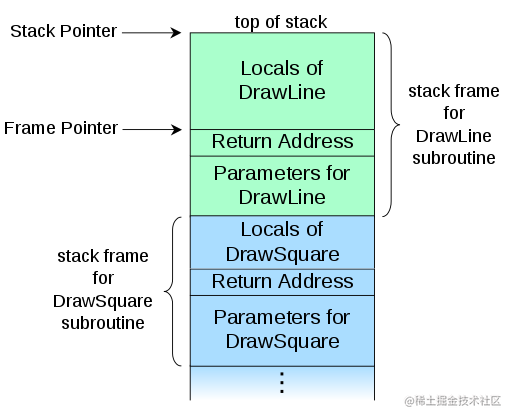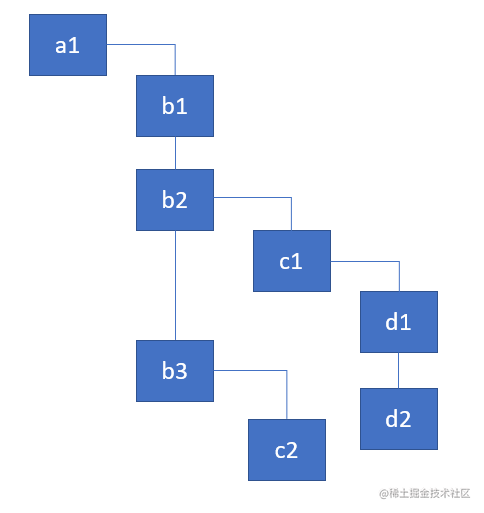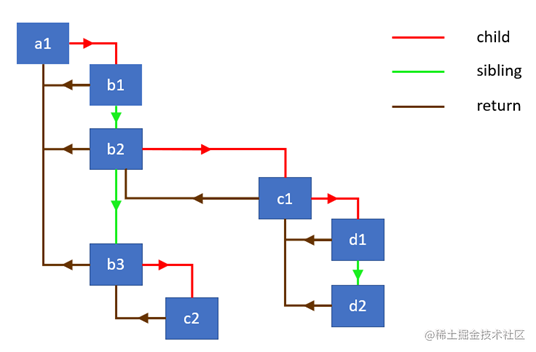# React 为何要使用链表遍历 Fiber 树

indepth.dev/the-how-and…

## 前置知识

Fiber 架构有两个主要的渲染阶段:

• reconciliation/render
• commit

• 更新 state 和 props
• 调用生命周期方法
• 检索当前组件的子组件
• 比对新老子组件
• 计算出需要在 commit 阶段被执行的 DOM 更新

``````requestIdleCallback((deadline)=>{
});

requestIdleCallback 在使用上其实是有局限性的, 不能 频繁地调用 它去实现平滑的 UI 渲染, 所以 React 团队 不得不实现他们自己的版本.

``````requestIdleCallback((deadline) => {
// while we have time, perform work for a part of the components tree
nextComponent = performWork(nextComponent);
}
});

## 什么是堆栈?

In computer science, a *call stack* is a stack data structure that stores information about the active subroutines of a computer program… the main reason for having call stack is *to keep track of the point* to which each active subroutine should return control when it finishes executing… A *call stack* is composed of *stack frames*… Each stack frame corresponds to a call to a subroutine which has not yet terminated with a *return*. For example, if a subroutine named `DrawLine` is currently running, having been called by a subroutine `DrawSquare`, the top part of the call stack might be laid out like in the adjacent picture.## 为什么栈和 React 有关?``````const a1 = {name: 'a1'};
const b1 = {name: 'b1'};
const b2 = {name: 'b2'};
const b3 = {name: 'b3'};
const c1 = {name: 'c1'};
const c2 = {name: 'c2'};
const d1 = {name: 'd1'};
const d2 = {name: 'd2'};

a1.render = () => [b1, b2, b3];
b1.render = () => [];
b2.render = () => [c1];
b3.render = () => [c2];
c1.render = () => [d1, d2];
c2.render = () => [];
d1.render = () => [];
d2.render = () => [];

React 需要遍历整个组件树并对每一个组件进行更新操作. 为了简化这个过程, 在更新每个组件的时候, 只会打印当前组件的 `name` 属性的值以及返回它的子组件. 下面是递归的实现方式.

## 递归遍历

``````walk(a1);

function walk(instance) {
doWork(instance);
const children = instance.render();
children.forEach(walk);
}

function doWork(o) {
console.log(o.name);
}

``````a1, b1, b2, c1, d1, d2, b3, c2

## 链表遍历

• child - 指向第一个子节点
• sibling - 指向第一个兄弟节点
• return - 指向父节点``````class Node {
constructor(instance) {
this.instance = instance;
this.child = null;
this.sibling = null;
this.return = null;
}
}

``````function link(parent, elements) {
if (elements === null) elements = [];

parent.child = elements.reduceRight((previous, current) => {
const node = new Node(current);
node.return = parent;
node.sibling = previous;
return node;
}, null);

return parent.child;
}

`link` 方法从后往前遍历节点列表, 将它们以单链表的形式连接. 函数最终返回一个指针, 指向列表中的第一个节点. 如下代码所示:

``````const children = [{name: 'b1'}, {name: 'b2'}];
const parent = new Node({name: 'a1'});

// the following two statements are true
console.log(child.instance.name === 'b1');
console.log(child.sibling.instance === children);

``````function doWork(node) {
console.log(node.instance.name);
const children = node.instance.render();
}

``````function walk(o) {
let root = o;
let current = o;

while (true) {
// perform work for a node, retrieve & link the children
let child = doWork(current);

// if there's a child, set it as the current active node
if (child) {
current = child;
continue;
}

// if we've returned to the top, exit the function
if (current === root) {
return;
}

// keep going up until we find the sibling
while (!current.sibling) {

// if we've returned to the top, exit the function
if (!current.return || current.return === root) {
return;
}

// set the parent as the current active node
current = current.return;
}

// if found, set the sibling as the current active node
current = current.sibling;
}
}Fiber 是调用栈的重新实现, 专为 React 组件设计. 你可以将一个 fiber 看作一个虚拟的栈帧.

``````function walk(o) {
let root = o;
let current = o;

while (true) {
...

current = child;
...

current = current.return;
...

current = current.sibling;
}
}

## React 事件循环

React 实现事件循环(`work loop`)的代码 在这里:

``````function workLoop(isYieldy) {
if (!isYieldy) {
// Flush work without yielding
while (nextUnitOfWork !== null) {
nextUnitOfWork = performUnitOfWork(nextUnitOfWork);
}
} else {
// Flush asynchronous work until the deadline runs out of time.
while (nextUnitOfWork !== null && !shouldYield()) {
nextUnitOfWork = performUnitOfWork(nextUnitOfWork);
}
}
}